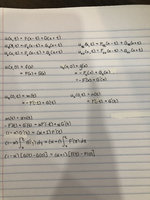# d'Alembert Formula

#### pdestud

##### New member
Use d'Alembert's solution of the wave equation to find the solution of

$$\displaystyle \frac{\partial^2 u}{\partial x^2} = \frac{\partial^2 u}{\partial t^2} \qquad (t \geq 0, x \geq 0)$$

$$\displaystyle \frac{\partial u(0,t)}{\partial t} = \alpha \frac{\partial u(0,t)}{\partial x} \qquad (t \geq 0)$$

$$\displaystyle u(x,0) = \phi_0 (x) \qquad (x \geq 0)$$

$$\displaystyle \frac{\partial u}{\partial t} (x, 0) = \phi_1 (x) \qquad (x \geq 0)$$

where $$\displaystyle \alpha \neq -1$$ is a constant and $$\displaystyle \phi_0 (x)$$ and $$\displaystyle \phi_1 (x)$$ are twice continuously differentiable for $$\displaystyle x > 0$$ and vanish near $$\displaystyle x = 0$$. Show that in general no solution exists when $$\displaystyle \alpha = -1$$.

#### Subhotosh Khan

##### Super Moderator
Staff member
Use d'Alembert's solution of the wave equation to find the solution of

$$\displaystyle \frac{\partial^2 u}{\partial x^2} = \frac{\partial^2 u}{\partial t^2} \qquad (t \geq 0, x \geq 0)$$

$$\displaystyle \frac{\partial u(0,t)}{\partial t} = \alpha \frac{\partial u(0,t)}{\partial x} \qquad (t \geq 0)$$

$$\displaystyle u(x,0) = \phi_0 (x) \qquad (x \geq 0)$$

$$\displaystyle \frac{\partial u}{\partial t} (x, 0) = \phi_1 (x) \qquad (x \geq 0)$$

where $$\displaystyle \alpha \neq -1$$ is a constant and $$\displaystyle \phi_0 (x)$$ and $$\displaystyle \phi_1 (x)$$ are twice continuously differentiable for $$\displaystyle x > 0$$ and vanish near $$\displaystyle x = 0$$. Show that in general no solution exists when $$\displaystyle \alpha = -1$$.

Please follow the rules of posting in this forum, as enunciated at:

Please review your post to make sure that you do not have any "typos".

How is this problem different from the "almost" duplicate problem you posted at:

•topsquark

#### pdestud

##### New member